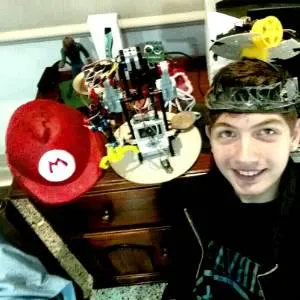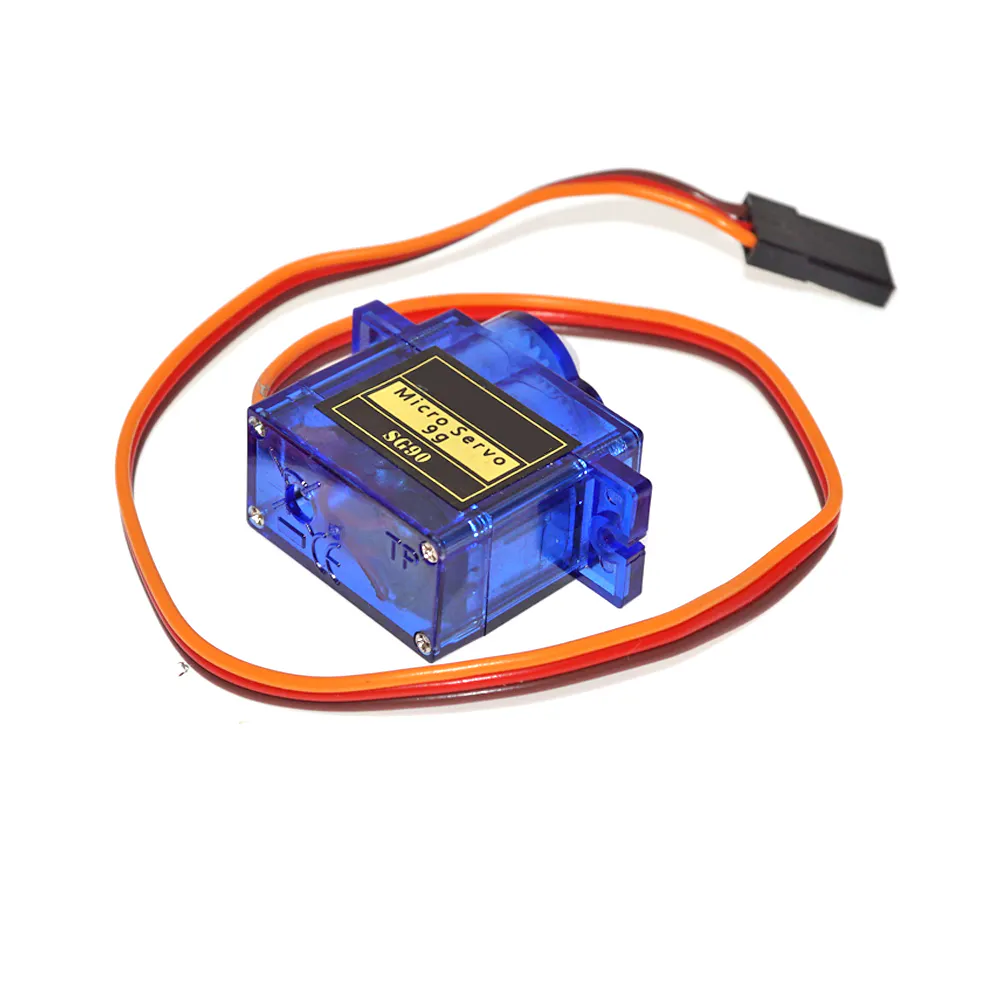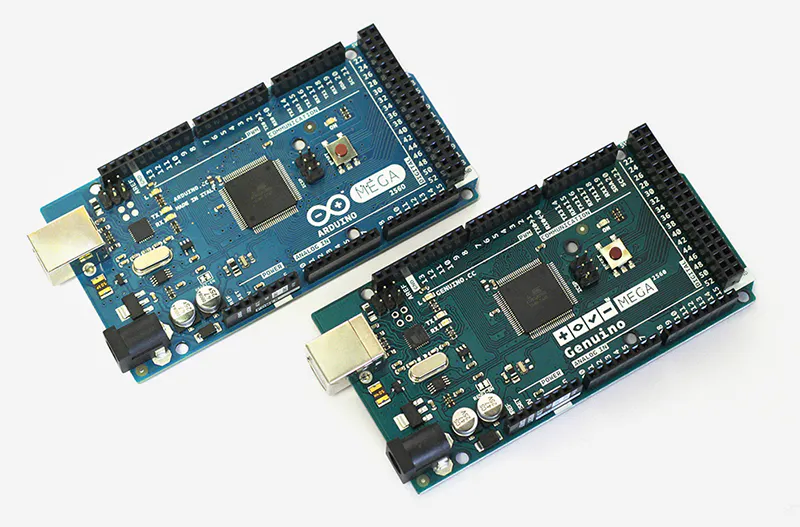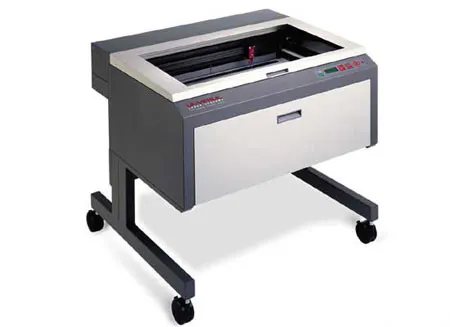# Mini Me, the Mini Humanoid Robot

Imagine being able to controll with your movements a mini version of yourself, well that is what this project is all about.

IntermediateWork in progress250## Things used in this project

### Hardware componentsSG90 Micro-servo motor
×10
 MG90 Servo Motor
×4Arduino Mega 2560 & Genuino Mega 2560
×1

### Software apps and online servicesArduino IDE

### Hand tools and fabrication machinesLaser cutter (generic)
 Handsaw (Generic)

## Schematics

### Main circuit

This is the main circuit, you will have to make this x2 with an Arduino Mega## Code

### Testing code

C/C++
This is the testing code i've beeing using for testing the strength of the servos
```#include <Servo.h>

int pos;
Servo pinza;
Servo muneca;
Servo codo;
Servo codoG;
Servo hombro;

void setup() {
pinza.attach(2);
muneca.attach(3);
codo.attach(4);
codoG.attach(5);
hombro.attach(6);

muneca.write(100);
codo.write(30);
codoG.write(100);
hombro.write(30);

}

void loop() {
//pinza
for (pos = 130; pos <= 180; pos += 1) { // goes from 0 degrees to 180 degrees
// in steps of 1 degree
pinza.write(pos);              // tell servo to go to position in variable 'pos'
delay(15);                       // waits 15ms for the servo to reach the position
}
for (pos = 180; pos >= 130; pos -= 1) { // goes from 180 degrees to 0 degrees
pinza.write(pos);              // tell servo to go to position in variable 'pos'
delay(15);                       // waits 15ms for the servo to reach the position
}

//muñeca
for (pos = 0; pos <= 180; pos += 1) { // goes from 0 degrees to 180 degrees
// in steps of 1 degree
muneca.write(pos);              // tell servo to go to position in variable 'pos'
delay(15);                       // waits 15ms for the servo to reach the position
}
for (pos = 180; pos >= 0; pos -= 1) { // goes from 180 degrees to 0 degrees
muneca.write(pos);              // tell servo to go to position in variable 'pos'
delay(15);                       // waits 15ms for the servo to reach the position
}
muneca.write(100);

//codo
for (pos = 0; pos <= 130; pos += 1) { // goes from 0 degrees to 180 degrees
// in steps of 1 degree
codo.write(pos);              // tell servo to go to position in variable 'pos'
delay(15);                       // waits 15ms for the servo to reach the position
}
for (pos = 130; pos >= 0; pos -= 1) { // goes from 180 degrees to 0 degrees
codo.write(pos);              // tell servo to go to position in variable 'pos'
delay(15);                       // waits 15ms for the servo to reach the position
}
codo.write(30);

//codoG
for (pos = 0; pos <= 180; pos += 1) { // goes from 0 degrees to 180 degrees
// in steps of 1 degree
codoG.write(pos);              // tell servo to go to position in variable 'pos'
delay(15);                       // waits 15ms for the servo to reach the position
}
for (pos = 180; pos >= 0; pos -= 1) { // goes from 180 degrees to 0 degrees
codoG.write(pos);              // tell servo to go to position in variable 'pos'
delay(15);                       // waits 15ms for the servo to reach the position
}
codoG.write(100);

//hombro
for (pos = 0; pos <= 180; pos += 1) { // goes from 0 degrees to 180 degrees
// in steps of 1 degree
hombro.write(pos);              // tell servo to go to position in variable 'pos'
delay(15);                       // waits 15ms for the servo to reach the position
}
for (pos = 180; pos >= 0; pos -= 1) { // goes from 180 degrees to 0 degrees
hombro.write(pos);              // tell servo to go to position in variable 'pos'
delay(15);                       // waits 15ms for the servo to reach the position
}

hombro.write(30);
}
```

## Credits

### AlguienMuyRobotico

1 project • 0 followers